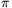Mathematical and Physical Journal
for High Schools
Issued by the MATFUND Foundation
 Already signed up? New to KöMaL?

#Problem C. 894. (March 2007)

C. 894. A hemispherical soup bowl has a volume of 8 litres. How much soup will fill the bowl to half its height?

(5 pont)

Deadline expired on April 16, 2007.

Sorry, the solution is available only in Hungarian. Google translation

Megoldás.ahonnan r3=12/dm3.

A gömbszelet térfogata:

V=/3.(r/2)2(3r-r/2)=/3(3 r3/4-r3/8)=2,5 dm3=2,5 l.

### Statistics:

 315 students sent a solution. 5 points: 208 students. 4 points: 55 students. 3 points: 18 students. 2 points: 9 students. 1 point: 1 student. 0 point: 2 students. Unfair, not evaluated: 22 solutionss.

Problems in Mathematics of KöMaL, March 2007